Next: 4.1.5 Vector-Valued Extension Up: 4.1 Principles of Fourier Previous: 4.1.3 Fourier Analysis of

## 4.1.4 Köhler Illumination of a Periodic Mask

The most important configuration of the illuminator in projection printing systems is due to Köhler [11, pp. 524-526]. In this method a converging lens commonly called condenser is positioned in front of the light source, whereby the light source lies in the focal plane of the lens (cf. Section 2.3.2). As illustrated in Figure 4.5 the rays from each source point then emerge from the condenser as parallel beams. This arrangement has the advantage that irregularities in the brightness distribution on the source do not cause irregularities in the intensity of the object illumination. Thus the quality of image composition severely depends on a careful and accurate set-up of the Köhler arrangement. For the mathematical description we assume an ideal set-up, so that the emerging beams from one source point form--after passage through the condenser--a plane wave. In complex phasor notation we get for the disturbance on the object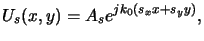(4.35)

with amplitude As and normalized wavevector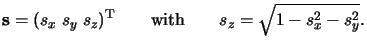(4.36)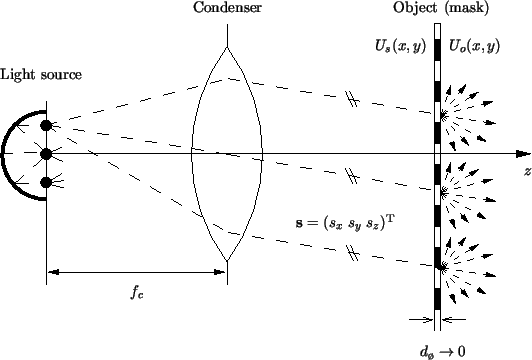In photolithography the object to be imaged is the photomask, whereby the pattern is either exclusively stored in the amplitude transmittance or in both amplitude and phase. Technological aspects of binary and phase-shifting masks were already discussed in Section 2.4. For aerial image simulation the mask is modeled to be infinitesimally thin with ideal transitions of the transmission characteristic, i.e., the mask thickness dø vanishes as shown in Figure 4.5. Depending on the mask type, the so-called transmission function t(x, y) is real-valued (zero or one) or complex-valued with module less than one. Similar to the phase transformation of the lens in (4.19) the field disturbance Us(x, y) incident on the mask is multiplied with the transmission function t(x, y) to obtain the object field immediately behind the mask, i.e.,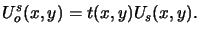(4.37)

This relation holds for the same reasons as discussed in connection with the Kirchhoff boundary conditions below (4.6) on page. For Köhler illumination we get with (4.38) for the object amplitude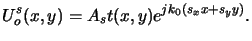(4.38)

Now we make a fundamental assumption that is used throughout all aerial image simulators: The photomask is periodic in both lateral directions x and y with periods a/M and b/M, respectively, i.e.,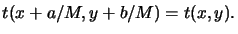(4.39)

The object periods are chosen as a/M and b/M, so that the image periods are a and b for a M : 1-projection system (cf. (4.37)). A direct consequence of this assumption is the possibility to represent the transmission function t(x, y) by a Fourier series, i.e.,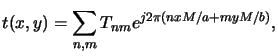(4.40)

whereby the Fourier coefficients are given by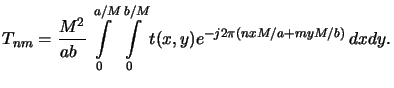(4.41)

Combining the two equations (4.41) and (4.43) yields for the object amplitude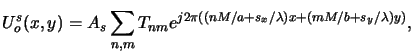(4.42)

whereby k0 = 2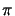/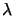has been inserted for the wavenumber. The object coordinates have to be scaled by the magnification M resulting in (cf. (4.35))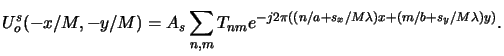(4.43)

Next we restrict the source point locations to a rectangular grid. Hence, only homogeneous plane waves from certain discrete orientations have to be considered further. We choose the spacing of the grid in the source plane in such a way that the lateral components of the normalized wavevector spq equal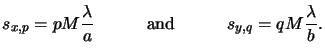(4.44)

The reason for this discretization will be explained later. Throughout aerial image simulation, it is more or less irrelevant although the resulting formulae are more compact. However, for an efficient exposure/bleaching simulation this specific choice is crucial as will be explained in Chapter 6.

Insertion of the discrete wavevectors (4.47) into (4.46) yields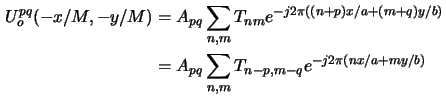for the scaled object amplitude due to one source point (p, q). Because of the periodicity of Uopq(- x/M, - y/M) its Fourier transform consists of a train of impulses occurring at the harmonically related frequencies n/a and m/b and writes to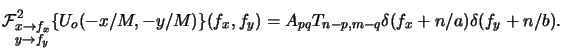(4.45)

Now we can easily determine the image spectrum from (4.37). Employing the equivalence property of the delta-functionk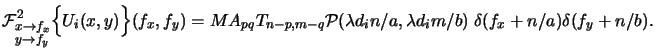(4.46)

Consequently, we obtain a Fourier series representation for the image such as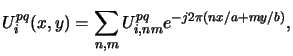(4.47)

whereby the Fourier coefficients are given by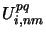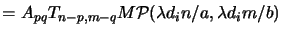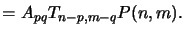(4.48)

As can be seen the discrete pupil function P(n, m) is related to the real physical pupil function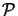(x, y) by P(n, m) = M(din/a,dim/b) and writes with (4.22) to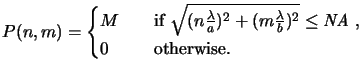(4.49)

The intensity Iipq(x, y) due to the illumination of the mask by one source point (p, q) is simply the squared module of the amplitude divided by two-times the free-space resistance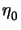,l i.e.,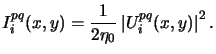(4.50)

#### Footnotes

... delta-functionk
The equivalence or filtering property of the delta function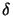(x) states that f (x) = h(x)(x - x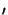) equals f (x) = h(x)(x - x).
...,l
The free-space resistance has a value of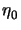=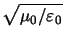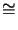377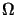.Next: 4.1.5 Vector-Valued Extension Up: 4.1 Principles of Fourier Previous: 4.1.3 Fourier Analysis of
Heinrich Kirchauer, Institute for Microelectronics, TU Vienna
1998-04-17